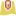# Historical Wildfire Analysis

Spatial wildfire occurrence data, referred to as the Fire Program Analysis fire-occurrence database (FPA FOD), for the United States, 1992-2015 was obtained from https://www.fs.usda.gov/rds/archive/Product/RDS-2013-0009.4/. The dataset contains data on:

• 1.88 Million US Wildfires
• 24 years of geo-referenced wildfire records

### Given this data, can we answer the following questions?

• Are wildfires increasing over time?
• Where are the fire hot spots?
• Can Machine Learning categorize the cause of the fire given the size, location and date?

That would give investigators a data driven way to prioritize cases for further investigation.

``````from arcgis import *
import pandas as pd
import numpy as np``````
``gis = GIS(profile='deldev.maps')``
``````from arcgis.features import GeoAccessor, GeoSeriesAccessor

wildfires_df = pd.DataFrame.spatial.from_featureclass(r'C:\demo\FPA_FOD_20170508.gdb\Fires')``````
``wildfires_df.shape``
`(1880465, 39)`
``````df = wildfires_df[['FIRE_YEAR', 'DISCOVERY_DATE', 'DISCOVERY_DOY',
'STAT_CAUSE_DESCR', 'FIRE_SIZE', 'STATE', 'FIPS_CODE', 'FIPS_NAME', 'SHAPE']]``````
``df.head()``
FIRE_YEARDISCOVERY_DATEDISCOVERY_DOYSTAT_CAUSE_DESCRFIRE_SIZESTATEFIPS_CODEFIPS_NAMESHAPE
020052005-02-0233Miscellaneous0.10CA063Plumas{'x': -121.00583333000003, 'y': 40.03694443999...
120042004-05-12133Lightning0.25CA061Placer{'x': -120.40444444000002, 'y': 38.93305556000...
220042004-05-31152Debris Burning0.10CA017El Dorado{'x': -120.73555555999997, 'y': 38.98416666999...
320042004-06-28180Lightning0.10CA003Alpine{'x': -119.91333333, 'y': 38.55916666999997, '...
420042004-06-28180Lightning0.10CA003Alpine{'x': -119.93305555999996, 'y': 38.55916666999...

### Exploratory Data Analysis

``````df['x'] = df.SHAPE.apply(lambda g: g['x'])
df['y'] = df.SHAPE.apply(lambda g: g['y'])
smdf = wildfires_df[['FIRE_YEAR', 'DISCOVERY_DATE', 'DISCOVERY_DOY',
'STAT_CAUSE_DESCR', 'FIRE_SIZE', 'STATE', 'FIPS_CODE', 'FIPS_NAME', 'x', 'y']]
smdf.to_pickle('fires.pkl')``````
``````pd.options.mode.chained_assignment = None

df['MONTH'] = pd.DatetimeIndex(df['DISCOVERY_DATE']).month
df['DAY_OF_WEEK'] = df['DISCOVERY_DATE'].dt.dayofweek
df_arson = df[df['STAT_CAUSE_DESCR']=='Arson']
dfa = df_arson['DAY_OF_WEEK'].value_counts()
df_lightning = df[df['STAT_CAUSE_DESCR']=='Lightning']
dfl = df_lightning['DAY_OF_WEEK'].value_counts()``````
``````%matplotlib inline
import matplotlib.pyplot as plt

plt.style.use('ggplot')
sorted_df = df.sort_values('FIRE_YEAR')
fire_freq = sorted_df.groupby('FIRE_YEAR').count()
x = np.asarray(fire_freq.axes)
y = np.asarray(df.groupby('FIRE_YEAR').size())``````
``````lyr = gis.content.search('Demographic Profile')
lyr``````
Demographic ProfileFeature Layer Collection by deldev

### Have wildfires become more frequent over time?

``````fig, ax = plt.subplots(figsize=(10,6))
ax.bar(x, y, color='coral')
plt.title('Number of fires by year', y=-0.2)
fit1 = np.polyfit(x, y, deg=1)
ax.plot(x, x*fit1 + fit1, color='teal', linewidth=4);``````### What are the causes of wildfire?

``distribution = df['STAT_CAUSE_DESCR'].value_counts()``
``````plt.figure(figsize=(6,6))
plt.title('Distribution by cause', y=-0.15)
plt.pie(distribution, labels=list(distribution.index[:-2]) + ['', '']);
plt.axis('equal');``````### Does the day of week matter?

``````ind = np.arange(7)
width = 0.35

fig, ax   = plt.subplots(figsize=(10,6))
arson     = ax.bar(ind, dfa.sort_index(), width, color='coral')
lightning = ax.bar(ind + width, dfl.sort_index(), width, color='teal')

ax.set_title('Wildfires by day of week', y=-0.15)
ax.set_xticklabels(('', 'Mon', 'Tues', 'Wed', 'Thu', 'Fri', 'Sat', 'Sun'))
ax.legend((arson, lightning), ('Arson', 'Lightning'), loc=2);``````## Mapping and Spatial Analysis

``wildfires = gis.content.import_data(wildfires_df)``
``````from arcgis.features.analysis import find_hot_spots
hotspots = find_hot_spots(wildfires, 20, 'Miles', output_name='Hot Spots US_Historical_Wildfires_20')``````
``hotspots = gis.content.get('8f9e60e114c749a9864e4adb9643d096')``
``````usmap = gis.map('United States')
usmap``````

## Can Machine Learning categorize the cause of the fire?

Since arson is a significant preventable cause for many wildfires, it would be instructive to see if Machine Learning techniques can be used to classify if a fire was caused due to arson given some basic data that is available when the fire is first discovered or when investigations begin. This can be framed as a binary classification problem.

Such a technique could give investigators a data driven way to prioritize cases for further investigation.

``````from sklearn.model_selection import train_test_split
from sklearn import tree, preprocessing
import sklearn.ensemble as ske
import pandas as pd
import matplotlib.pyplot as plt

%matplotlib inline
%config IPCompleter.greedy=True``````
``````df = pd.read_pickle('fires.pkl')

df['MONTH'] = pd.DatetimeIndex(df['DISCOVERY_DATE']).month
df['DAY_OF_WEEK'] = df['DISCOVERY_DATE'].dt.dayofweek

ca_fires = df[df.STATE=='CA']
ca_fires['ARSON'] = ca_fires['STAT_CAUSE_DESCR'].apply(lambda x: 1 if x == 'Arson' else 0)

pdf = ca_fires[ ['FIRE_YEAR', 'DISCOVERY_DOY', 'FIPS_CODE', 'FIRE_SIZE', 'x', 'y', 'MONTH', 'DAY_OF_WEEK', 'ARSON'] ]
pdf = pdf.dropna()``````

### Feature Engineering: Use geoenrichment to add features

``````analysis_variables = [
'TOTPOP_CY',  # 2016 Population: Total Population (Esri)
'HHPOP_CY',   # 2016 Household Population (Esri)
'FAMPOP_CY',  # 2016 Family Population (Esri)
'DIVINDX_CY', # 2016 Diversity Index (Esri)
'TOTHH_CY',   # 2016 Total Households (Esri)
'AVGHHSZ_CY', # 2016 Average Household Size (Esri)

'MALES_CY',   # 2016 Gender: Male Population (Esri)
'FEMALES_CY', # 2016 Gender: Female Population (Esri)

'MEDAGE_CY',  # 2016 Age: Median Age (Esri)

'AVGFMSZ_CY', # 2016 Income: Average Family Size (Esri)
'MEDHINC_CY', # 2016 Income: Median Household Income (Esri)
'AVGHINC_CY', # 2016 Income: Average Household Income (Esri)

'EDUCBASECY', # 2016 Educational Attainment Base (Esri)
'NOHS_CY',    # 2016 Education: Less than 9th Grade (Esri)
'SOMEHS_CY',  # 2016 Education: 9-12th Grade/No Diploma (Esri)
'HSGRAD_CY',  # 2016 Education: High School Diploma (Esri)
'GED_CY',     # 2016 Education: GED/Alternative Credential (Esri)
'SMCOLL_CY',  # 2016 Education: Some College/No Degree (Esri)
'ASSCDEG_CY', # 2016 Education: Associate's Degree (Esri)
'BACHDEG_CY', # 2016 Education: Bachelor's Degree (Esri)
]``````
``````from arcgis.geoenrichment import *

usa = Country.get('US')
counties = usa.subgeographies.states['California'].counties

enrich_df = enrich(list(counties.values()), analysis_variables=analysis_variables)

pdf['StdGeographyID'] = '06' + pdf['FIPS_CODE']
merged = pd.merge(pdf, enrich_df, on='StdGeographyID')
cols = ['FIRE_YEAR', 'DISCOVERY_DOY', 'FIPS_CODE', 'FIRE_SIZE', 'x', 'y', 'MONTH', 'DAY_OF_WEEK', 'ARSON'] + analysis_variables
mdf = merged[cols]

02005330630.1-121.00583340.03694422019292...4527566596148024479093153415480316722048
120051530632.3-120.45111139.81722263019292...4527566596148024479093153415480316722048
220052340630.5-121.14750039.87222280019292...4527566596148024479093153415480316722048
320052410630.1-120.90388939.96222280019292...4527566596148024479093153415480316722048
420052420631.0-120.70666740.05694481019292...4527566596148024479093153415480316722048

5 rows × 29 columns

``````m = gis.map('California')
m ``````
``lyr = gis.content.import_data(enrich_df, title='Demographic Profile')``
``m.add_layer(lyr, {'renderer':'ClassedColorRenderer', 'field':'AVGHINC_CY'})``

### Fit Random Forest classifier and check accuracy

``````X = mdf.drop(['ARSON'], axis=1).values
y = mdf['ARSON'].values
X_train, X_test, y_train, y_test = train_test_split(X,y,test_size=0.3, random_state=42)

clf_rf = ske.RandomForestClassifier(n_estimators=200)
clf_rf = clf_rf.fit(X_train, y_train)

print(clf_rf.score(X_test, y_test))``````
```0.9212070907689571
```

Summary: Given some basic data, available when a fire is first discovered, it is possible to predict with over 90% accuracy if the fire was the result of arson.

Citations

Short, Karen C. 2017. Spatial wildfire occurrence data for the United States, 1992-2015 [FPA_FOD_20170508]. 4th Edition. Fort Collins, CO: Forest Service Research Data Archive. https://doi.org/10.2737/RDS-2013-0009.4